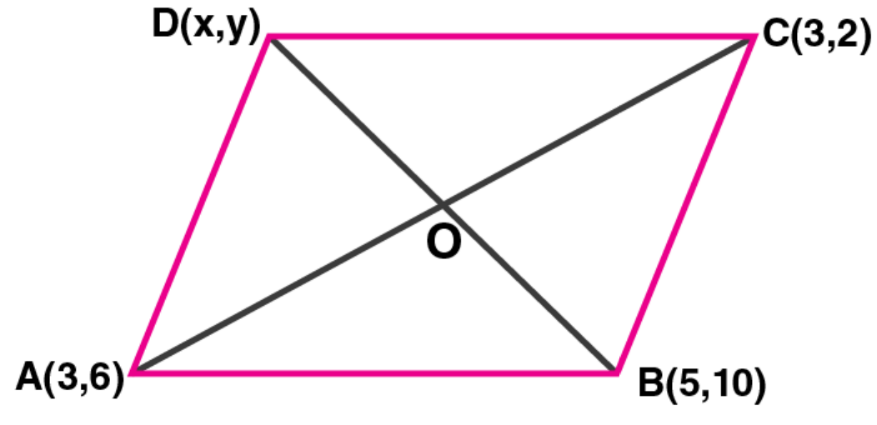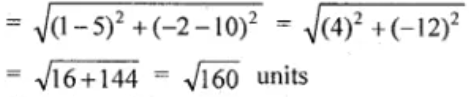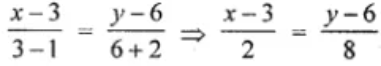Newbie

# Three vertices of a parallelogram ABCD taken in order are A (3, 6), B (5, 10) and C (3, 2) find: (i) the coordinates of the fourth vertex D. (ii) length of diagonal BD. (iii) equation of side AB of the parallelogram ABCD.

• 1

An Important Question of class 10 Based on Equation of a Straight Line Chapter of M.L Aggarwal for ICSE BOARD.
Here three vertices of a parallelogram is given
So, you have to find the coordinates of the fourth vertex , length of diagonal and equation of a side.
This is the Question Number 29, Exercise 12.1 of M.L Aggarwal.

Share

1.Given, the three vertices of a parallelogram ABCD taken in order are A (3, 6), B (5, 10) and C (3, 2)

(i) We know that the diagonals of a parallelogram bisect each other.

Let (x, y) be the co-ordinates of D

Hence, we have

Mid-point of diagonal AC = ((3 + 3)/2, (6 + 2)/2) = (3, 4)

Mid-point of diagonal BD = ((5 + x)/2, (10 + y)/2)And, these two should be the same

On equating we get,

(5 + x)/2 = 3 and (10 + y)/2 = 4

5 + x = 6 and 10 + y = 8

x = 1 and y = -2

Thus, the co-ordinates of D = (1, -2)

(ii) Length of diagonal BD(iii) Equation of the side joining A (3, 6) and D (1, -2) is given by4 (x – 3) = y – 6

4x – 12 = y – 6

4x – y = 6

Thus, the equation of the side joining A (3, 6) and D (1, -2) is 4x – y = 6.

• 1